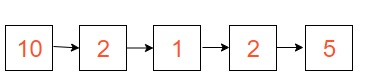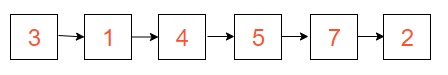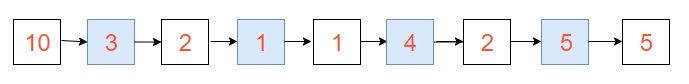# Merge a linked list into another linked list at alternate positions in Java

We are given two data structures as linked list Let’s say, List_1 and List_2. The task is to merge the elements of linked list ‘List_2’ into the linked list ‘List_1’ at an alternate position and if we are left with the elements which can't be merged into ‘List_1’ then it will be printed as the ‘List_2’ remaining elements.

## For Example-:

In

List_1 =List_2 =Out − Merged List is-:Explanation − we are given with two lists i.e. List_1 and List_2. We had merged the possible elements of list_2 into List_1 at alternate positions. So, elements after merging in List_1 are 10- >3->2->1->1->4->2->5->5 and in List_2 are 7->2.

In

List_1 = 11 -> 12 -> 13

List_2 = 14 -> 15 -> 16 -> 17 -> 18

Out −Merged List is -: 11 -> 14 -> 12 -> 15 -> 13

Explanation −we are given with two lists i.e. List_1 and List_2. We had merged the possible elements of list_2 into List_1 at alternate positions. So, elements after merging in List_1 are 11 - > 14 -> 12 -> 15 -> 13 and in List_2 are 16->17->18.

## Approach used in the below program is as follows −

• Create a head node that will point to the first node of a linked list.

• Create a class of Node to create the linked list which will have value and next as data members. Define a default constructor as Node(int val) and set value as val and next as NULL.

• Create an object as new_node and pass updated_value to the default constructor.

• Inside a function in mergeList(TutorialPoint list)

• Create an object as n1_curr and set to head and n2_curr to list.head

• Create an object as n1_next and n2_next

• Start While as n1_curr != null AND n2_curr != null. Inside the While set n1_next to n1_curr.next, n2_next to n2_curr.next, n2_curr.next to n1_next, n1_curr.next to n2_curr, n1_curr to n1_next and n2_curr to n2_next

• Inside the main() method

• Create an object as TutorialPoint list_1 to new TutorialPoint() and TutorialPoint list_2 to new TutorialPoint()

• Call the mergeList method to merge the elements of list_2 to list_1 as list_1.mergeList(list_2)

• Print the final list as an output.

## Example

public class TutorialPoint{
class Node{
int value;
Node next;
Node(int val){
value = val;
next = null;
}
}
Node new_node = new Node(updated_value);
}
void mergeList(TutorialPoint list){
Node n1_next, n2_next;

while (n1_curr != null && n2_curr != null){
n1_next = n1_curr.next;
n2_next = n2_curr.next;

n2_curr.next = n1_next;
n1_curr.next = n2_curr;

n1_curr = n1_next;
n2_curr = n2_next;
}
}
public static void main(String args[]){
TutorialPoint list_1 = new TutorialPoint();
TutorialPoint list_2 = new TutorialPoint();
list_1.mergeList(list_2);
System.out.println("Merged list is:");
while (temp != null){
System.out.print(temp.value + " ");
temp = temp.next;
}
System.out.println();
}
}

## Output

If we run the above code it will generate the following Output

Merged list is:
11 14 12 15 13 16